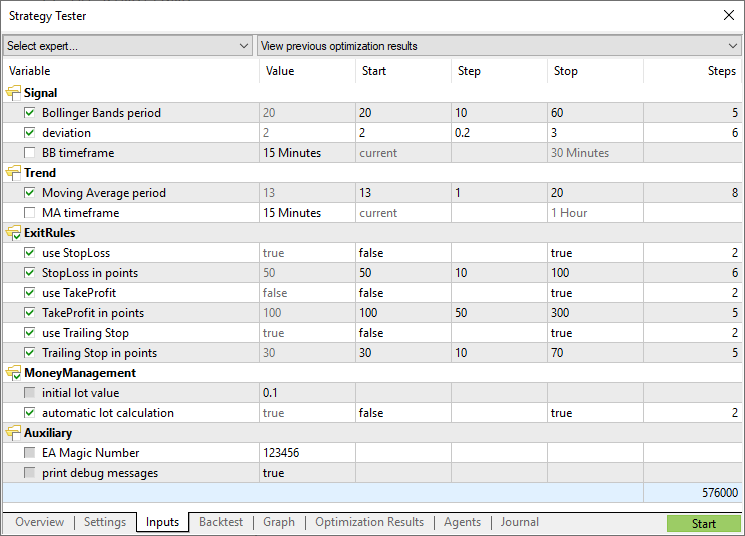# 输入变量

 //--- 输入参量 input int            MA_Period=13; input int            MA_Shift=0; input ENUM_MA_METHOD MA_Method=MODE_SMMA;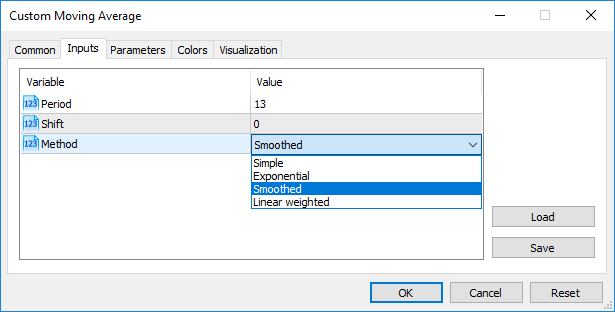//--- 输入参量 input int            InpMAPeriod=13;         // 平滑周期 input int            InpMAShift=0;           // 水平移动行 input ENUM_MA_METHOD InpMAMethod=MODE_SMMA;  // 平滑函数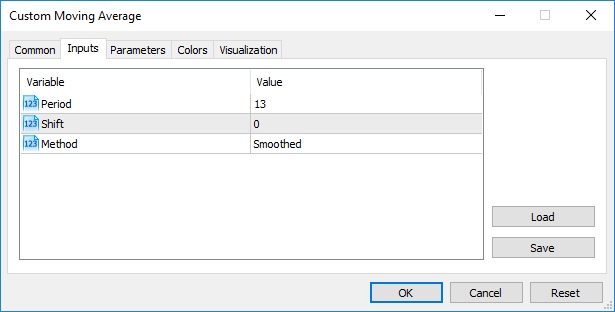• 参数值由"parameter_name=parameter_value"字符串表示（考虑'='），
• 最大表示长度为255个字符（total_length_max=255或254个字符，不包括'='），
• parameter_name_length字符串参数的最大长度 = 63个字符。

 parameter_value_length=total_length_max-parameter_name_length=254-parameter_name_length

### MQL5程序访问自定义指标时传送参数 #

iCustom()函数访问自定义指标。其名后，参数要与该指标声明的输入变量严格一致。如果参数少于变量，缺失的参数要在变量声明时用特殊值填满。

### 计数作为输入参数

MQL5提供得嵌入计数和用户指定的变量都可以用作输入变量（mql5程序的输入参数）。例如，可以创建dayOfWeek计数，描述一周的天数，使用输入变量指定某一天，不是数字，用更通常的方法。

 #property script_show_inputs //--- 一周内每天 enum dayOfWeek    {    S=0,     // 周日    M=1,     // 周一    T=2,     // 周二    W=3,     // 周三    Th=4,    // 周四    Fr=5,    // 周五,    St=6,    // 周六   }; //--- 输入参量 input dayOfWeek swapday=W;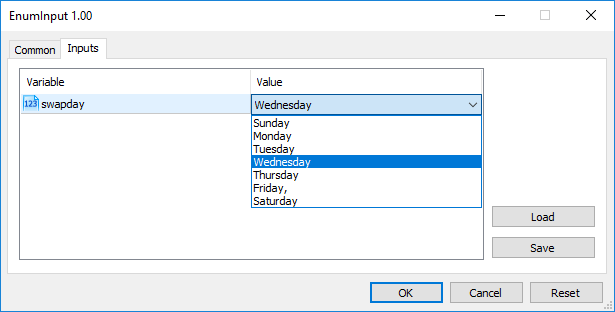## Sinput变量修饰符 #

 sinput       int layers=6;   // 层数

 static input int layers=6;   // 层数

Sinput修饰符声明的变量是MQL5程序的一个输入参数。当启动程序时可以更改该参数的值。然而，这个变量不用于输入参数的优化。换句话说，当寻找适合指定条件的最好的一组参数时不会枚举它的值。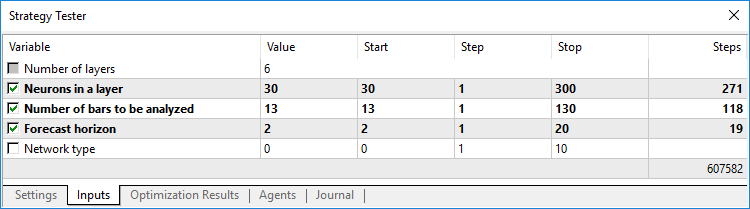## 排列输入参数 #

 input group           "Group name" input int             variable1 = ... input double          variable2 = ... input double          variable3= ...

 input group           "Group name #1" input int             group1_var1 = ... input double          group1_var2 = ... input double          group1_var3 = ...   input group           "Group name #2 input int             group2_var1 = ... input double          group2_var2 = ... input double          group2_var3 = ...

 input group           "Signal" input int             ExtBBPeriod   = 20;       // 布林带周期 input double          ExtBBDeviation= 2.0;      // 偏差 input ENUM_TIMEFRAMES ExtSignalTF=PERIOD_M15;   // BB 时间周期   input group           "Trend" input int             ExtMAPeriod   = 13;       // 移动平均线周期 input ENUM_TIMEFRAMES ExtTrendTF=PERIOD_M15;    // MA 时间周期   input group           "ExitRules" input bool            ExtUseSL      = true;     // 使用“止损” input int             Ext_SL_Points = 50;       // “止损”点数 input bool            ExtUseTP      = false;    // 使用“止盈” input int             Ext_TP_Points = 100;      // “止盈”点数 input bool            ExtUseTS      = true;     // 使用“追踪止损” input int             Ext_TS_Points = 30;       // “追踪止损”点数   input group           "MoneyManagement" sinput double         ExtInitialLot = 0.1;      // 初始手数值 input bool            ExtUseAutoLot = true;     // 自动手数计算   input group           "Auxiliary" sinput int            ExtMagicNumber = 123456;  // EA幻数 sinput bool           ExtDebugMessage= true;    // 打印调试信息